#### Introduction

Calorimétrie Adiabatique de Réaction (ARC)The method describing isothermal and adiabatic test procedures used to detect thermally exothermic decomposition reactions.Accelerating Rate Calorimetry (ARC®) is a method for studying Worst-Case ScenarioRelated to a chemical reactor, a worst-case scenario is the situation where temperature and/or pressure production caused by the reaction runs out of control.worst-case scenarios and Thermal runawayA thermal runaway is the situation where a chemical reactor is out of control with respect to temperature and/or pressure production caused by the chemical reaction itself. Simulation of a thermal runaway is usually carried out using a calorimeter device according to accelerated rate calorimetry (ARC).thermal runaway reactions. In contrast to other caloric techniques such as reaction calorimetry, combustion calorimetry or differential scanning calorimetry (DSC), ARC®-type equipment allows for an AdiabatiqueAdiabatic describes a system or measurement mode without any heat exchange with the surroundings. This mode can be realized using a calorimeter device according to the method of accelerating rate calorimetry (ARC). The main purpose of such a device is to study scenarios and thermal runaway reactions. A short description of the adiabatic mode is “no heat in – no heat out”.adiabatic sample environment. Adiabaticity is essential in order to observe the most violent reaction progress possible. Decomposition reactions, which are of particular interest in this context, produce heat and pressure since the reactions are usually heavily exothermic and are forming Réaction de DécompositionA decomposition reaction is a thermally induced reaction of a chemical compound forming solid and/or gaseous products. decomposition gases. The adiabatic sample environment is realized inside the ARC®-type calorimeter via a set of heaters surrounding the sample compartment and a clever temperature control regime. One aim is to detect the temperature at which the self-decomposition of a sample or a sample mixture starts. Another goal is to prevent any exchange of heat between the sample and its surrounding once the exothermic decomposition reaction has started. As soon as the Self-heating rateA special kind of calorimeter device is used in order to detect the self-heating rate of a substance. The related method is called accelerating rate calorimetry (ARC). self-heating rate exceeds a certain threshold (which is usually in the range of 0.02 K/min), all heaters surrounding the sample will track the sample temperature. Without heat exchange, there will be no heat loss to the surrounding, and if no heat dissipates, the entire heat of reaction remains inside the sample, thus increasing the sample temperature. The higher the sample temperature, the faster the rate of reaction will be. Such an experiment not only delivers the starting temperature of the decomposition reaction under quasi-isothermal conditions, it also allows for determination of the maximum temperature rise and the maximum pressure rise under adiabatic conditions.

#### The PHI-Factor (φ) or “Thermal Inertia“

From the two signals measured, temperature and pressure, the maximum rate can be calculated and predictions are usually made for the temperature at which the investigated reaction takes a minimum of twenty-four hours to reach its maximum temperature development rate, the time-to-maximum rate (TMR24h).

An essential parameter for the test scenario is what is known as the PHI-factorThe PHI-factor (Φ) is equivalent to the thermal inertia. Both describe the ratio of mass and specific heat capacity of a sample or sample mixture compared to that of the vessel or sample container. PHI-factor (φ). It gives the ratio of mass and specific heat of the sample to the sample vessel, where ΔTad is the temperature rise under adiabatic conditions, ΔTobs is the observed temperature rise under given conditions, m is the mass, Specific Heat Capacity (cp)Heat capacity is a material-specific physical quantity, determined by the amount of heat supplied to specimen, divided by the resulting temperature increase. The specific heat capacity is related to a unit mass of the specimen.cp is the Specific Heat Capacity (cp)Heat capacity is a material-specific physical quantity, determined by the amount of heat supplied to specimen, divided by the resulting temperature increase. The specific heat capacity is related to a unit mass of the specimen.specific heat capacity, s is the sample and v is the vessel .

The φ-factor, also known as Thermal inertiaThe thermal inertia is equivalent to the  PHI-factor. Both describe the ratio of mass and specific heat capacity of a sample or sample mixture compared to that of the vessel or sample container.thermal inertia, is better the closer it gets to 1, which means in the ideal case that the test results are defined through the sample and not through the influence of the vessel. On the other hand, the above-mentioned equation points out that the ratio of sample-to-vessel mass is somehow yielded by the reactivity of the sample itself, along with the maximum volume of the sample container and the materials available for the vessels. In order to show how these parameters will influence the φ-factor, table 1 summarizes φ-factors calculated for two samples (organic peroxides and hydrogen peroxide), two vessel materials (stainless steel and titanium) and for a realistic variety of sample masses.

Table 1: Calculated Ф-factors for various measurement conditions

The correlation of sample mass and calculated φ-factor mentioned above is additionally shown in figure 1. Since the Specific Heat Capacity (cp)Heat capacity is a material-specific physical quantity, determined by the amount of heat supplied to specimen, divided by the resulting temperature increase. The specific heat capacity is related to a unit mass of the specimen.specific heat capacity of the sample to be investigated along with the Specific Heat Capacity (cp)Heat capacity is a material-specific physical quantity, determined by the amount of heat supplied to specimen, divided by the resulting temperature increase. The specific heat capacity is related to a unit mass of the specimen.specific heat capacity of the vessel material is usually given, the only parameter available for changing the φ-factor is the sample mass.

Increasing the sample mass can bring the φ-factor closer to 1, but there might be limitations to the volume of the vessel as well as limitations associated with the equipment itself. It is imperative to keep in mind the pressure range, temperature range and maximum tracking rate of the ARC®-type calorimeter used in order to not exceed one of them; otherwise, data might no longer be meaningful. It can be seen from figure 1 that, due to its total volume of 2.6 ml, the stainless steel vessel (figure 3) is limited to a sample mass of less than 2.0 g. Since vessels are usually not filled more than half, the expected φ-factor is between 2 and 4, depending on the Specific Heat Capacity (cp)Heat capacity is a material-specific physical quantity, determined by the amount of heat supplied to specimen, divided by the resulting temperature increase. The specific heat capacity is related to a unit mass of the specimen.specific heat capacity of the sample itself. Only with 1.5 mg hydrogen peroxide, which has a relatively high Specific Heat Capacity (cp)Heat capacity is a material-specific physical quantity, determined by the amount of heat supplied to specimen, divided by the resulting temperature increase. The specific heat capacity is related to a unit mass of the specimen.specific heat capacity, can a φ-factor of better than 2 be established. Even when using a titanium vessel offering 8.6 ml in volume, sample masses of more than 3.0 g and φ-factors in the range of 1.5 are somehow difficult to realize.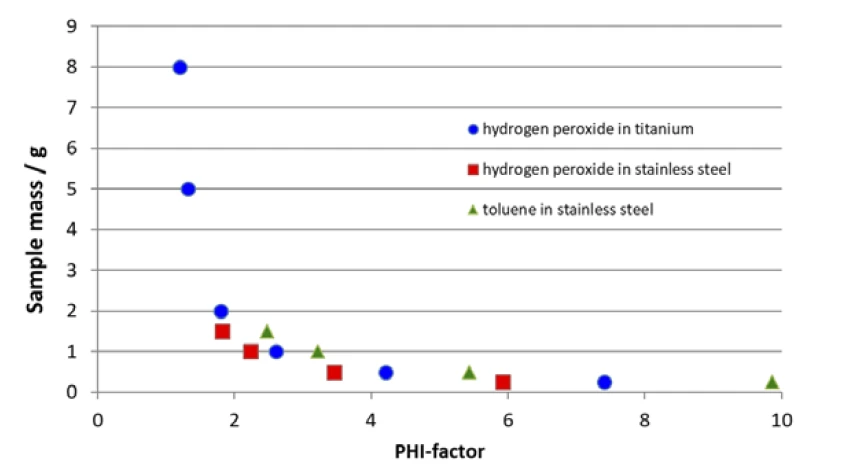1) Correlation of the sample mass and φ-factor for different samples and vessel materials according to equation 1. The associated titanium vessel is shown in figure 2; the associated stainless steel container in figure 3.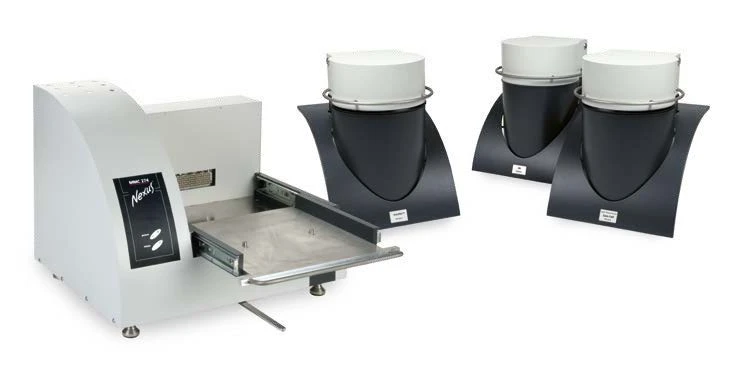4) NETZSCH Multiple Module Calorimeter (MMC)A multiple mode calorimeter device consisting of a base unit and exchangeable modules. One module is prepared for accelerating rate calorimetry (ARC), the ARC-Module. A second one is used for scanning tests (Scanning Module) and a third one is related to battery testing for coin cells (Coin Cell Module).MMC 274 Nexus® Multiple Module Calorimeter

All samples which exhibit Thermal hazard potentialThe likeliness or the risk of an individual chemical or a chemical mixture to undergo an exothermic self-decomposition reaction under uncontrolled circumstances (thermal runaway).thermal hazard potential are also characterized by increased risk with regard to handling in a laboratory environment. From a safety point of view, it is of course far better to handle risky samples in small amounts. In consideration of the above-discussed limitations, a dilemma ensues. The lower the φ-factor, the more meaningful the results should be. This, however, would require larger sample amounts. Yet decreasing the sample mass to address the safety issues will increase the φ-factor. In order to overcome this dilemma, the patented VariPhi® was used inside an ARC® Module of the Multiple Module Calorimeter (MMC)A multiple mode calorimeter device consisting of a base unit and exchangeable modules. One module is prepared for accelerating rate calorimetry (ARC), the ARC-Module. A second one is used for scanning tests (Scanning Module) and a third one is related to battery testing for coin cells (Coin Cell Module).MMC 274 Nexus®.

#### The Multiple Module Calorimeter (MMC 274 Nexus®)

The Multiple Module Calorimeter (MMC)A multiple mode calorimeter device consisting of a base unit and exchangeable modules. One module is prepared for accelerating rate calorimetry (ARC), the ARC-Module. A second one is used for scanning tests (Scanning Module) and a third one is related to battery testing for coin cells (Coin Cell Module).MMC 274 Nexus® Multiple Module Calorimeter (figure 4) offers three different measurement modules . The Coin-Cell Module is specialized for the investigation of batteries and the Scanning ModuleA calorimeter module being part of the Multipe Module Calorimeter (MMC) allowing for scanning test of a sample. This procedure can serve as a screening test in order to detect a thermal hazard potential within a reasonably short measurement time.Scanning Module [3, 4] can be used to evaluate caloric data from a single heating run. The ARC® Module (figure 5) can be used for thermal hazard studies and was employed for the results presented in this work.

#### Test Substance: Hydrogen Peroxide Solution

Hydrogen peroxide (H2O2) thermally decomposes into water and oxygen. This decomposition reaction can be initiated thermally and is heavily exothermic. This is why hydrogen peroxide is usually handled as an aqueous solution of up to 35%. In terms of thermal safety studies, it is an ideal substance since it forms water and oxygen during decomposition and this makes the cleaning and reuse of vessels quite convenient.

#### The ARC® Module with VariPhi®

Figure 5 shows the setup of the MMC’s ARC® Module. The sample container is placed inside the calorimeter compartment and the sample temperature is detected via a thermocouple clamped directly to the outer wall of the sample container. The vessel itself is connected via a feed-through to a pressure gauge. Right in the center of this setup, the internal heater, called VariPhi®, is placed inside the sample.

This patented VariPhi® heater is the solution to the abovedescribed dilemma. On the one hand, it can be used for a screening run in order to quickly detect whether or not an unknown sample exhibits hazardous potential. In this case, constant power would be supplied to the VariPhi® heater. Along with the resulting heating rate, a heat-flow signal can be calculated in order to distinguish between endothermic and exothermic sample effects. On the other hand, the VariPhi® heater can also be used to partially or fully compensate for the influence of the sample vessel (φ-factor; eq. 1). In this case, the VariPhi® heater applies the amount of heat to the sample which would usually be lost by warming up the sample container. Since the sample is the warmest part during a selfheating decomposition reaction, heat would be lost to warm up the vessel prior to being detected via the thermocouple which is clamped outside the vessel (figure 5). According to equation 1, the φ-factor can be either partially or completely compensated to achieve the ideal conditions with respect to the φ-factor. This way, it is possible to adjust the φ-factor to a value which reflects the real conditions of a reactor or it can be adjusted to φ = 1 in order to study Worst-Case ScenarioRelated to a chemical reactor, a worst-case scenario is the situation where temperature and/or pressure production caused by the reaction runs out of control.worst-case scenarios. The required power input for compensation is given by the mass and specific heat capacity of the vessel.

If a thermal hazard screening test has detected self-heating and pressure build-up (figure 6), it is imperative to carry out an additional thermal-runaway test. The results for such a Heat-Wait-Search (HWS)Heat-Wait-Search is a measurement mode used in calorimeter devices according to accelerating rate calorimetry (ARC).heat-wait-search (Heat-Wait-Search (HWS)Heat-Wait-Search is a measurement mode used in calorimeter devices according to accelerating rate calorimetry (ARC).HWS) test are depicted in figure 7. It compares the differences between the compensated measurement results (red curve) and non-compensated ones (black curve). The measurement conditions are summarized in table 2.

In contrast to the scanning test, the corresponding Heat-Wait-Search (HWS)Heat-Wait-Search is a measurement mode used in calorimeter devices according to accelerating rate calorimetry (ARC).heat-wait-search test of hydrogen peroxide detects the beginning of the self-heating already at 90°C (figure 7, black curve). The maximum Self-heating rateA special kind of calorimeter device is used in order to detect the self-heating rate of a substance. The related method is called accelerating rate calorimetry (ARC). self-heating rate was detected to be 0.08 K/min together with an temperature increase of 26.8 K (ΔTobs). The observed temperature increase is evaluated by subtracting the onset temperature (Tstart, beginning of the ExothermiqueA sample transition or a reaction is exothermic if heat is generated.exothermal event) from the final temperature of the exothermal event (Tfinal) .

The above-discussed measurement results, depicted by the black curve in figure 7, are carried out without making use of the internal heater, called VariPhi®; the associated φ-factor is 3.14. When employing the VariPhi® for the same sample setup and using its power to compensate for the mass and specific heat capacity of the vessel (φ = 1), the measured temperature increase was determined to be 64.8 K (red curve, figure 7). This nicely confirms the expectation of a significant increase in both ΔTobs and the speed of reaction. The lower the φ-factor, the less heat is lost in warming up the sample vessel; furthermore, all the heat of reaction can remain inside the sample vessel to speed up the self-heating reactions. The dashed line in figure 7 confirms a Self-heating rateA special kind of calorimeter device is used in order to detect the self-heating rate of a substance. The related method is called accelerating rate calorimetry (ARC). self-heating rate almost ten times higher for the measurement using VariPhi® (red curve, figure 7) than for the non-compensated measurement. These results demonstrate the enormous impact of the φ-factor with respect to the expected hazard potential of chemical reactions.

If VariPhi® is not available, measurements can usually not be carried out with low-φ conditions due to limitations caused by material properties of the sample container, maximum sample amount, expected pressure, etc. In this case, ASTM E1981 – 81(2012) suggests the following approximation for ideal measurement conditions.

The “delta T ideal” value is calculated as per equation 3 during data evaluation in NETZSCH Proteus® software. The non-compensated result (black curve in figure 7) indicates a “ ΔTobs” of 26.8 K and a φ-factor of 2.56. The assumption for a measurement result under ideal conditions (φ = 1) expects “ ΔTideal” to be 68.6 K. This assumption made via equation 3 is close to the measurement result of 64.8 K yielded by using the VariPhi® heater (red curve in figure 7).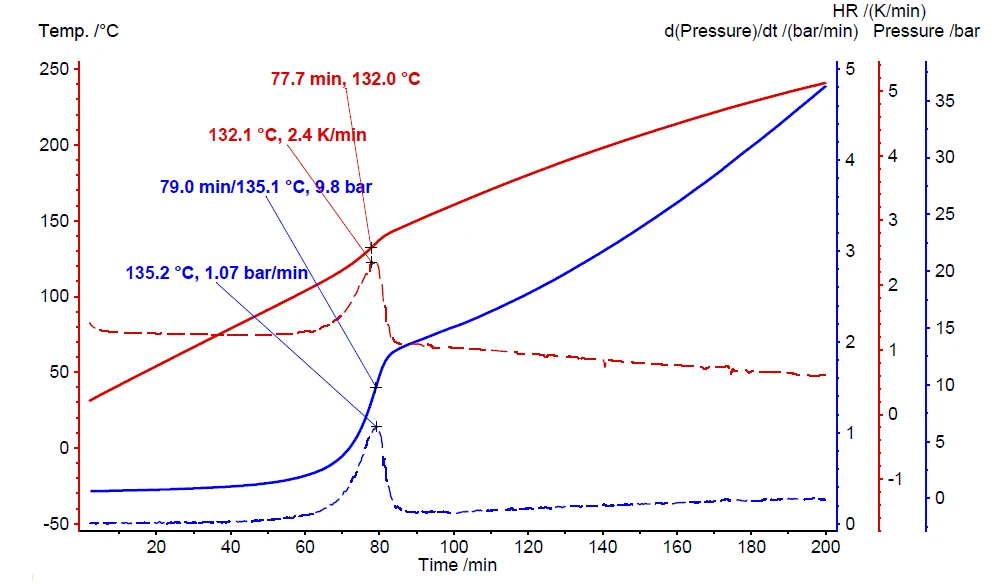6) Measurement results for screening of hydrogen peroxide with pressure (blue), pressure rate (dashed blue), temperature (red) and temperature rate (dashed red)

Table 2: Measurement conditions for scanning (figure 6) and the heat-wait-seach tests (figure 7)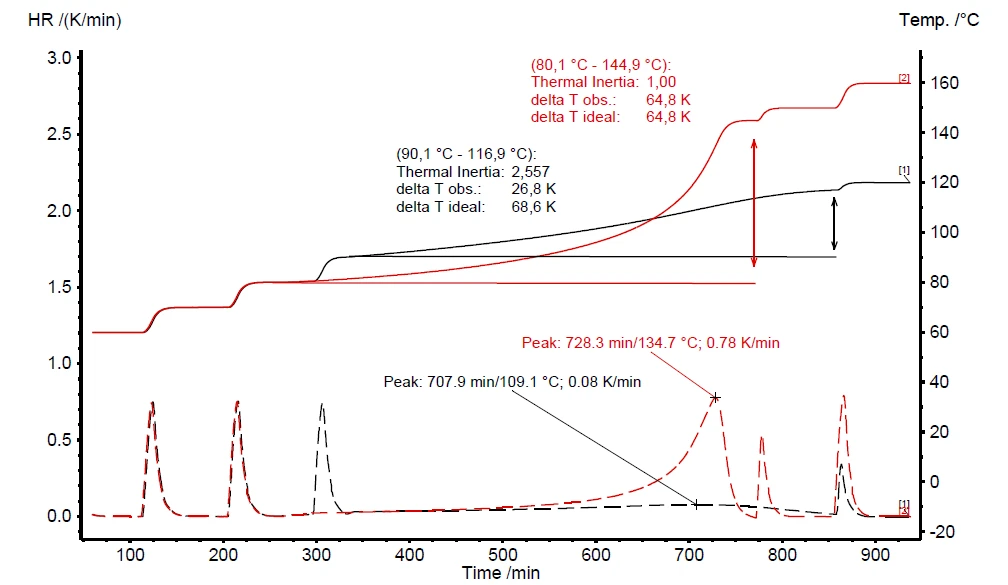7) Measurement results for a Heat-Wait-Search (HWS)Heat-Wait-Search is a measurement mode used in calorimeter devices according to accelerating rate calorimetry (ARC).heat-wait-search (Heat-Wait-Search (HWS)Heat-Wait-Search is a measurement mode used in calorimeter devices according to accelerating rate calorimetry (ARC).HWS) test on hydrogen peroxide with compensation of the φ-factor (red) and without (black)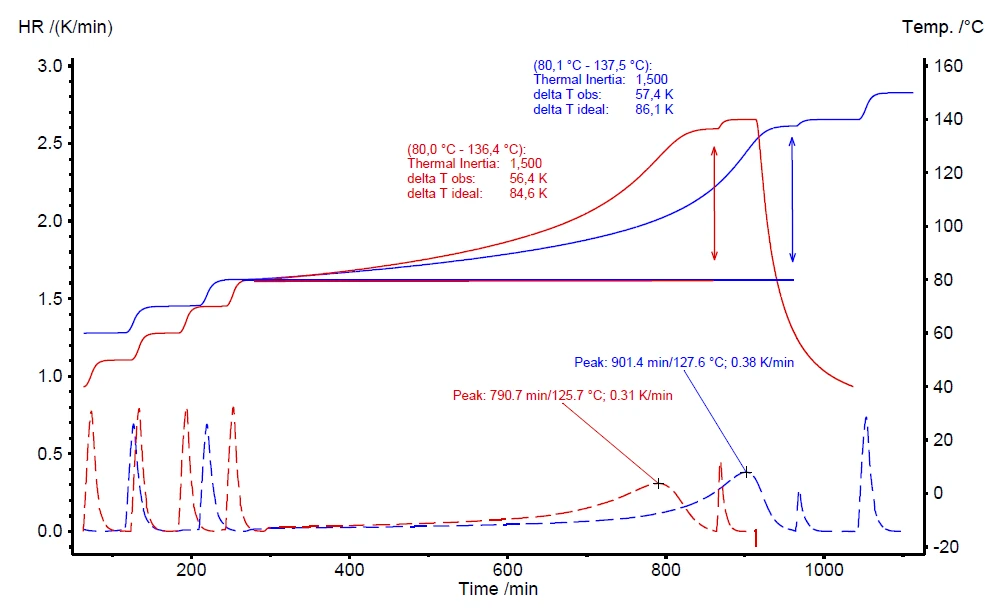8) Comparison of two Heat-Wait-Search (HWS)Heat-Wait-Search is a measurement mode used in calorimeter devices according to accelerating rate calorimetry (ARC).heat-wait-search (Heat-Wait-Search (HWS)Heat-Wait-Search is a measurement mode used in calorimeter devices according to accelerating rate calorimetry (ARC).HWS) tests with different measurement conditions, both compensated to φ = 1.5

A further advantage of the VariPhi® heater is to compensate for the φ-factor to improve the comparability of different measurement conditions. Figure 8 compares two measurements on different amounts of hydrogen peroxide. The red curve represents a measurement on 0.500 g of H2O2 (φ = 4.21) and the blue measurement was carried out using 1.00 g (φ = 2.60). Due to the different sample masses, the φ-factors are significantly different: 4.21 and 2.60, respectively. The VariPhi® heater was employed to compensate both measurements to φ = 1.5. The evaluated results are very similar for the two measurements, including the onset temperature (Tstart), the Self-heating rateA special kind of calorimeter device is used in order to detect the self-heating rate of a substance. The related method is called accelerating rate calorimetry (ARC). self-heating rate (HR) and the observed temperature increase (ΔTobs).

#### Conclusion

The decomposition reaction of hydrogen peroxide (H2O2) was investigated as a test scenario to demonstrate the use of an additional heater inside ARC®-type equipment. The patented VariPhi® heater can be employed to compensate the test setup to either a real world φ-factor or to the ideal value of φ = 1. This setup of compensation for heat loss allows low-φ measurements on even small sample amounts. From a safety point of view, the ability to vary the φ-factor turns out to be a great advantage for laboratories testing the hazardous potential of chemicals and reaction mixtures.

#### Literature

1. 
ASTM E1981 – 98(2012), “Standard Guide forAssessing Thermal Stability of Materials by Methods of Accelerating Rate Calorimetry”, ASTM International, 100 Barr Harbor Drive, PO Box C700, West Conshocken, PA19428-2959.
2. 
E. Füglein, S. Schmölzer, “Epoxy Curing Investigatedby Means of DSC 214 Polyma and MMC 274 Nexus®®“, NETZSCH Application Note 130, 2019E. Füglein, S. Schmölzer, “Epoxy Curing Investigatedby Means of DSC 214 Polyma and MMC 274 Nexus®®“, NETZSCH Application Note 130, 2019
3. 
E. Füglein, “Hazard Potential of Decomposition Reactions Using the Example of Hydrogen Peroxide (H2O2)”, NETZSCH Application Note 131, 2019
4. 
E. Füglein, “Screening of Hydrogen Peroxide Solutionsby Means of Scanning Tests and ARC® Tests®, NETZSCH Application Note 132, 2019